## 2.4.1 Electronic Band Structure of Graphene

Within the tight-binding method the two-dimensional energy dispersion relations of graphene can be calculated by solving the eigen-value problem for a HAMILTONian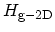associated with the two carbon atoms in the graphene unit cell . In the SLATER-KOSTER scheme one gets2.1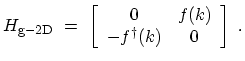(2.3)

where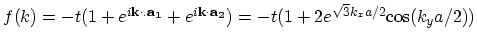and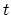is the nearest neighbor C-C tight binding overlap energy2.2 . Solution of the secular equation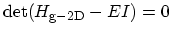leads to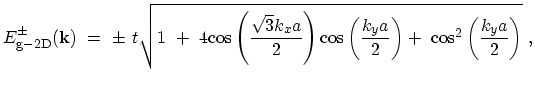(2.4)

where the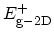and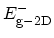correspond to the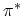and the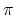energy bands, respectively. Figure 2.6 shows the electronic energy dispersion relations for graphene as a function of the two-dimensional wave-vector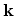in the hexagonal BRILLOUIN zone.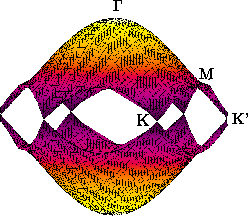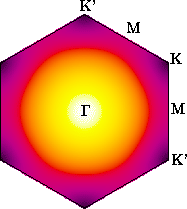M. Pourfath: Numerical Study of Quantum Transport in Carbon Nanotube-Based Transistors